# Focal Length Calculators

This is an online calculator to find the focal length of a lens. Just enter the object size, image size, and object distance to get the magnification, angle of view, and focal length value.

• mm
• mm
• mm

### Result

• Magnification
• Focal Length of the Lens
mm
• Effective Focal Length
mm
• Angle of View
Degrees

Thin Lens are those lenses whose thickness can be neglected when compared to the lengths of the radii of curvature of its two refracting surfaces, and to the distances of the objects and images from it.

Focal Length of a thin lens is the distance from the center of the lens to the principal focus of the lens.

Image formation in a lens takes place by the refraction of light at its two bounding surfaces. Each surface of the lens acts as an image-forming component and contributes to the final image formed by the lens.Consider a thin convex lens XY with an optical center at C. Let the refractive index of the lens µ2 and that of the surrounding medium be µ1. The centers of curvature of the two refracting surfaces of the lens are C1 and C2 and let the corresponding radii of curvature be R1 and R2 respectively. Consider a point object 'O' placed on the principal axis of the lens.

A ray of light OA strikes the first surface at A and is refracted in the direction 'BI1'. This ray is further refracted by the second surface in the direction of 'BI' and meets the ray which passes undeviated through the principal axis at 'I'. So, the final image is formed at 'I' after the refraction by the two surfaces of the lens.

Considering the refraction at the spherical surfaces we can derive an equation for the image formation. The basic concept is that the image formed by the first refracting surface acts as a virtual object for the second refracting surface. By applying the Gauss Formula which is a geometrical method to describe the behavior of light, we can write,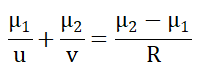The first surface XAY forms a real point-image I1. So in the above equation, v can be replaced by v1 and R by R1. Using sign convention, the distances measured to the left of the principal axis is negative. So, here u is negative and the above equation can be rewritten as,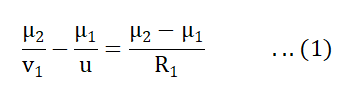From the figure, the light leaving the first surface would form an image I1 if the second surface was absent. With the second surface, I1 becomes a virtual object for the second surface and it forms an image at I, which is the final image. The equation for the final image formation can be written using the Gauss formula. Here we take u = v and R = R2, thus we get,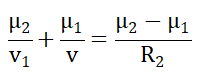Applying the sign convention, we get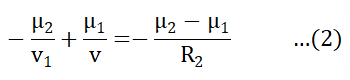Adding (1) and (2), and simplifying we get,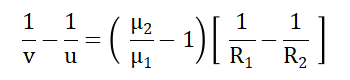Taking the surrounding medium as air, µ1=1 and also taking the refractive index of the lens as µ, we get,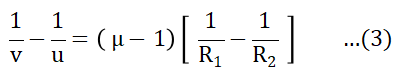If we consider the object at infinity, the image will be formed at the principal focus of the lens. So put u=∞ and v=f in the above equation and can be rewritten as,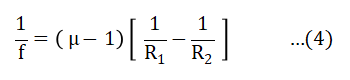This equation is known as the Lens Maker’s Formula.

Comparing equations (3) and (4), we get,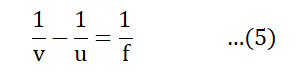This equation is the Gauss’ Formula for a lens.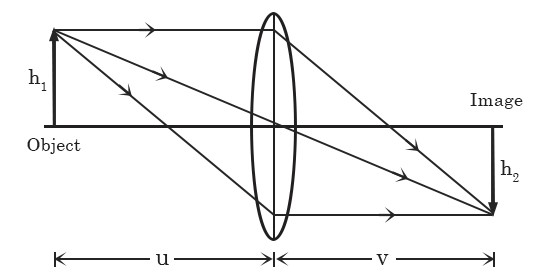Lenses are used to form a magnified image of an object. Magnification is defined as,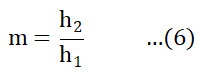where h1 and h2 are the object height and image height respectively.

By referring to figure 2, we take the refractive indices on either side of the lens as the same, then we have,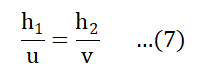So comparing (6) and (7), we get,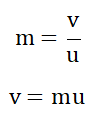Substituting this value of v in equation (5) and simplifying, we get,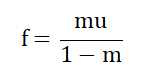In the case of a thin lens, where the lens-to-object distance is comparable to the focal length, we need to take into account the magnification factor, so we can write the effective focal length as,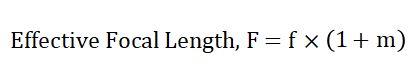Angle of View is the angular measure of how much of the area can be captured by the lens. It is defined in terms of the image size and the effective focal length,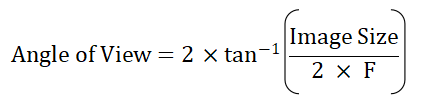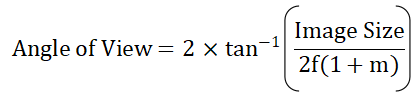This is an online calculator to find the focal length of a mirror. Just enter the object size, image size, and object distance to get the magnification, angle of view, and focal length value.

• mm
• mm
• mm

### Result

• Magnification
• Focal Length of the Mirror
mm
• Effective Focal Length
mm
• Angle of View
Degrees

Mirror is any surface that redirects light rays, thereby forming an image of the object. They may be plane or curved. Image formation by mirrors involves only the law of reflection.

Focal Length of a mirror is the distance between the vertex of the mirror and the focal point.Consider the image formation by a spherical mirror. Figure shows a concave mirror with a radius of curvature R. The center of curvature of the surface is at C and the vertex of the mirror is at P. The line OP is the optic axis. Point O is an object lying on the optic axis and we assume that the distance OP is greater than R.

The ray from O at the angle α with the axis strikes the mirror at Q and is reflected. The incident and reflected rays make equal angle θ with the normal CQ (law of reflection). A second ray from O, passing through C strikes the mirror normally and is reflected back on itself. The two reflected rays intersect at I forming a real image of O.

In a triangle, the exterior angle is equal to the sum of the two opposite interior angles. Applying this in ∆OQC,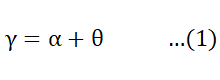Similarly in ∆OQL,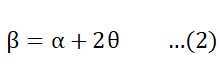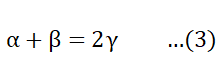Let h be the height of the point Q above the optic axis and let δ be the short distance BP.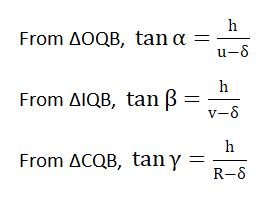According to paraxial approximation, for small angles, α, β, Ƴ, tan α can be replaced by α and so on. So we get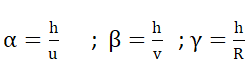Substituting these values in (3), we get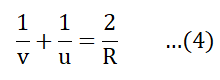This is known as the Spherical Mirror Equation.

If the object is taken at a very large distance from the mirror (u=∞), then the incoming rays are almost parallel, then the above equation becomes,The point at which the incident parallel rays converge is called the focal point and the distance from the vertex to the focal point is called the focal length denoted by f. So focal length is the image distance when the object is at infinity. Thus, v = f and f = R/2

Thus (4) can be rewritten as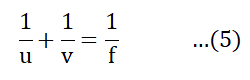This is known as the Gauss formula for Spherical Mirrors.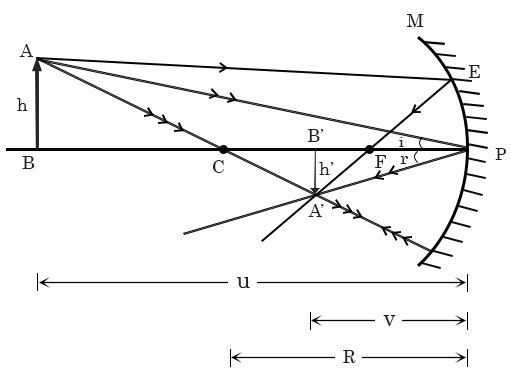The ratio of dimensions of the final image formed by an optical system to the corresponding dimension of the object is defined as the magnification. Here the object and image have different sizes and they have opposite orientations. Then from the figure, we can write,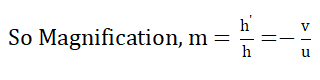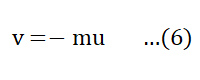Thus rewriting (5) using (6), we get,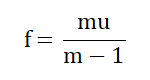In the case of a mirror, where the mirror-to-object distance is comparable to the focal length, we need to consider the magnification factor, hence the effective focal length can be written as,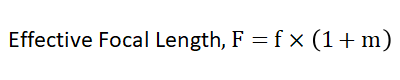Angle of View is the angle subtended at the image of the eye by the mirror. It is defined in terms of the image size and the effective focal length,This is an online calculator to find the focal length of a thick lens. Just enter the radius of curvature, refractive index, and thickness to get the nominal power, effective power, and focal length value.

• mm
• mm
• mm

### Result

• Nominal Power
• Effective Power
• Effective Focal Length
mm

Thick lens is a physically large lens whose spherical surfaces are separated by a distance. In other words, it is a lens whose thickness cannot be neglected when compared to its focal length.

Focal length is the distance between the focal point and the optical center of a lens. It is the measure of the ability of a lens to converge or diverge a ray of light. The plane perpendicular to the principal axis of the lens and passing through its focal point is known as the focal plane.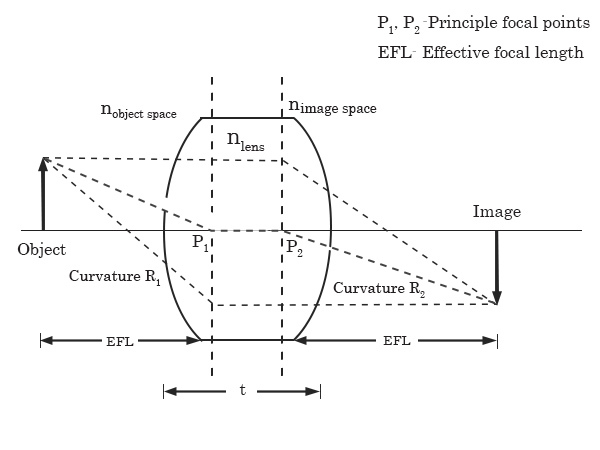Focal Point is the point to which a set of rays parallel to the principal axis is set to converge (in the case of a convex lens) or appear to diverge (in the case of a concave lens).

Optical Center is a point on the principal axis for which the rays passing through it are not deviated by the lens. Any ray passing through the optic center emerges in a direction parallel to the incident ray, i.e. the ray emerges undeviated.

Principle Axis is the line joining the centers of curvature of the two curved surfaces of a lens.

A lens has two curved surfaces and each surface has a curvature.

Radius of Curvature of a lens is the radius of that circle of which the curvatures of the lens is a part.

Power of a lens is the measure of its ability to produce convergence of a parallel beam of light. A convex lens has a converging effect and thus its power is taken as positive, but a concave lens produces divergence and so its power is taken as negative. The unit in which the power of a lens is measured is called Dioptre. Mathematically,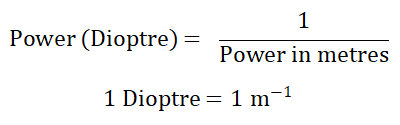In the case of a thick lens, the optical power of the surfaces depends on the refractive index of the lens. Thus the power output of both surfaces will be different.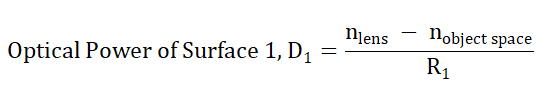Where nlens is the refractive index of the lens, nobject space is the refractive index of the medium in which the object is kept and R1 is the radius of curvature of surface 1.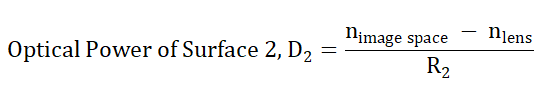nimage space is the refractive index of the medium in which the image is formed, and R2 is the radius of curvature of surface 2.

Nominal Power of a lens is algebraically the sum of optical powers of each surface. It is denoted as 'DNP'.

Where, DNP = D1 + D2

Since the thickness is non-negligible, the Effective Power is not just simply the sum of the individual powers, but it depends on the thickness of the lens. The equation for the effective power of a lens is given by the Gullstrand Equation as,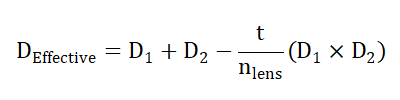where 't' is the thickness of the lens.

In such a lens where the power depends on the thickness of the lens, the effective focal length cannot be taken as the sum of individual foci of the two surfaces. So, we define Effective Focal Length as the reciprocal of the effective power of the lens, showing the thickness dependence of the lens.